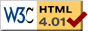### Journée-séminaire de combinatoire

#### (équipe CALIN du LIPN, université Paris-Nord, Villetaneuse)

Le 10 mars 2020 à 10h30 en B107, Gérard Duchamp nous parlera de : Identities between entire functions and their Hopf algebraic counterpart (1/2)

Résumé : Euler's reflection identity between inverse Gamma functions (with center zero) suggest that there are symmetrisation procedures that amount to the lacunarization of the exponents in exponentials. We start from, precisely, the lacunarization of the exponent in the Eulerian identity $$\dfrac1{\Gamma(1+z)} =\exp\biggl(\gamma z-\sum_{n\ge2}\zeta(n)(-z)^n/n\biggr)$$ and obtain, of course, a family of new entire function (with real coefficients).
What is surprising, is that these "new" entire functions are the images of algebraically independent characters of the stuffle algebra. Algebraic independence of the characters is obtained via general theorems like Radford's lemma on characters, "Kleene stars of the plane" phenomena and the Lie-theoretic setting of the BTT (basic triangle theorem).

The seminar is thought as interactive and a walk along a route through (Hopf) algebra(s), complex analysis, functional analysis and combinatorics on words.

 Dernière modification : Monday 24 January 2022Contact pour cette page : Cyril.Banderier at lipn.univ-paris13.fr